1. /
2. CBSE
3. /
4. Class 04
5. /
6. Mathematics
7. /
8. NCERT Solutions for Class...

# NCERT Solutions for Class 4 Maths Play With Patterns

NCERT solutions for Class 4 Mathematics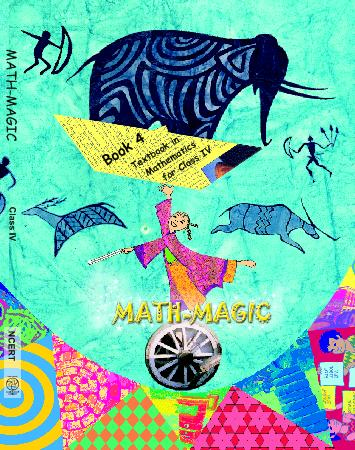## NCERT Solutions for Class 4 Maths Play With Patterns

Chapter -10 Class 4 Maths Play With Patterns

### 1. We can also make patterns with numbers and letters. Below are a few examples. Can you take them forward?

Ans. By following the given pattern, we can take them forward as under: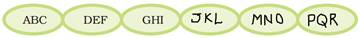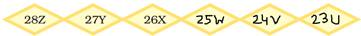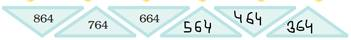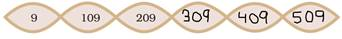### 2. Now write your own number patterns.

Ans.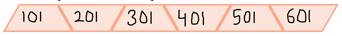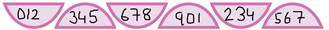### 3. Now you try writing the letters – A, B, C in the box so that no letter comes twice in any line.

Ans. After writing the letters- A, B, C in the box so that no letter comes twice in any line, the filled up box is as shown: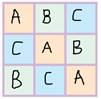### 4. Now you fill these stars. Use numbers 1 – 9 and the rule that the numbers in each line add up to 15.

Ans. The numbers 1 – 9 can be filled in these stars so that the number on each line add up to 15.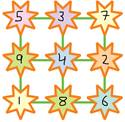### 5. Now use number 1 – 6 to make your own magic triangle.

Ans. The number 1- 6 can be filled in these square boxes as shown so that the numbers each side add up to 10: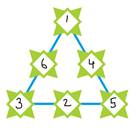### 6. Using the same rule, complete these number towers.

Ans. (i)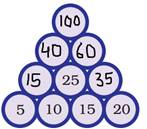5 + 10 = 15

15 + 20 = 35

15 + 25 = 40

25 + 35 = 60

40 + 60 = 100

(ii)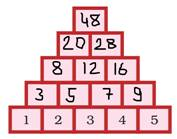1 + 2 = 3

2 + 3 = 5

3 + 4 = 7

4 + 5 = 9

3 + 5= 8

5 + 7 = 12

7 + 9 = 16

8 + 12 = 20

12 + 16 = 28

### 7. Now you write any number and the three numbers after that. Make a pattern using the rule.

Ans. Writing any number and the three numbers after that, we get the numbers recorded in the box. Using the above rule, we find that we get the same sum.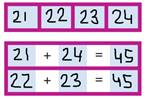### 8. Now, you try to make such a pattern with 5 numbers in order.

Ans.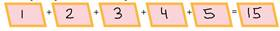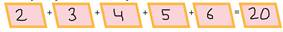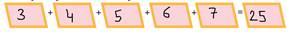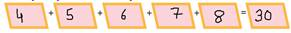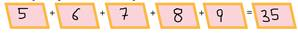### 9. Does the sum grows by 5 each time?

Ans. Yes, the sum grows by 5 each time.

### 10. Complete this list of letters and numbers to help you.

Ans. The complete list of letters and numbers is as under: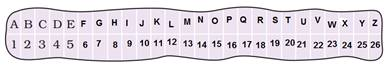### 11. Teenu wants to write to his friend “Good Morning”. What will he write by using the same rule.

Ans. Teenu will write: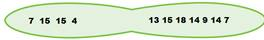### 12. If we change the rule and write 1 in place of ‘B’, 3 rd in place of ‘B’ , 3 in place of ‘D’ and so on, then how will be write “Let Us Dance”?

Ans. It can be written as: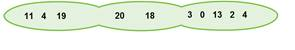### 13. Secret’s Messages:

(a) What was Kahuli’s secret message?

Ans. (a) His message is – WE ARE STUMPS.

(b) What did Shablu and Jaggu write?

Ans. IFMMP! IPX BSF ZPV? Means HELLO! HOW ARE YOU?

GJOF, UIBOL ZPV means FINE, THANK YOU.

XJMM ZPV QMBZ XJUI NF? means WILL YOU PLAY WITH ME?

(c) Use the same rule to write- “Meet me on the moon”.

Ans. (c) It can be written as NFFU NF PO UIF NPPO.

### 14. Now, Anisha is playing with this card. Draw what it will look upside down.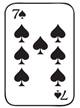Ans. It will look like: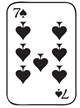### 15. Have you ever seen a floor with the tiles of shapes (given in book)?

Ans. Yes, I have seen a floor with tiles of these shapes.

## NCERT solutions for Class 4 Mathematics Chapter 10 Play With Patterns

Building with BricksView Solutions
Long and ShortView Solutions
A trip to BhopalView Solutions
Tick Tick TickView Solutions
The Way the World LooksView Solutions
The Junk SellerView Solutions
Jugs and MugsView Solutions
Carts and WheelsView Solutions
Halves and QuartersView Solutions
Play With PatternsView Solutions
Table and SharesView Solutions
How Heavy How LightView Solutions
Fields And FencesView Solutions
Smart ChartsView Solutions

NCERT Solutions Class 4 Maths Play With Patterns PDF (Download) Free from myCBSEguide app and myCBSEguide website. Ncert solution class 4 Maths includes text book solutions from Class 4 Maths Book . NCERT Solutions for CBSE Class  Maths have total 14 chapters. 4 Maths NCERT Solutions in PDF for free Download on our website. Ncert Maths class 4 solutions PDF and Maths ncert class 4 PDF solutions with latest modifications and as per the latest CBSE syllabus are only available in myCBSEguide.

## CBSE app for Students

To download NCERT Solutions for Class 4 Maths, EVS Hindi English, Maths Science do check myCBSEguide app or website. myCBSEguide provides sample papers with solution, test papers for chapter-wise practice, NCERT solutions, NCERT Exemplar solutions, quick revision notes for ready reference, CBSE guess papers and CBSE important question papers. Sample Paper all are made available through the best app for CBSE students and myCBSEguide website.Test Generator

Create question paper PDF and online tests with your own name & logo in minutes.myCBSEguide

Question Bank, Mock Tests, Exam Papers, NCERT Solutions, Sample Papers, Notes

### 6 thoughts on “NCERT Solutions for Class 4 Maths Play With Patterns”

1. Nice

2. I Like this soluson

3. Very nice answer good working. You are already maintend in study. Very good.

4. Hello

5. Thnx for helping us .. answers here r describe so nicely…so thnx a lot

6. It’s very easy to understand